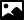## Data Category em_classes

### GeneralCategory name
em_classes
Required in PDB entries
no
Category group membership
em_group  em_reconstruction_group  em_single_particle_group
Used in current PDB entries
No

### Category Relationship DiagramsView full category relationship diagram including all dictionary data categories

### Category Description```    Data items in the EM_CLASSES category record details
about the particle classification.
Particle classification involves grouping images that are similar,
and separating images that are distinct. In practical use, this means
that experimental projections that have the same orientation (shape)
are placed within the same category for later averaging. In this case,
orientation means that the particles are showing the same face to the
viewer and the only difference between them is that they can be rotated
by some angle in the plane of the image. The experimental projections
might also be shifted relative to each other, but the centering of
the experimental projections is often done before classification.

is this required?
E(e1,e2,e3) = E(w,h,i)

cos(i)cos(h)cos(w)-sin(i)sin(h)    cos(i)cos(h)sin(w)+sin(i)sin(h)  -cos(i)sin(h)
-sin(i)cos(h)cos(w)-cos(i)sin(h)    sin(i)cos(h)sin(w)+cos(i)sin(h)   sin(i)sin(h)
sin(h)cos(w)                       sin(h)sin(w)                      cos(h)

```